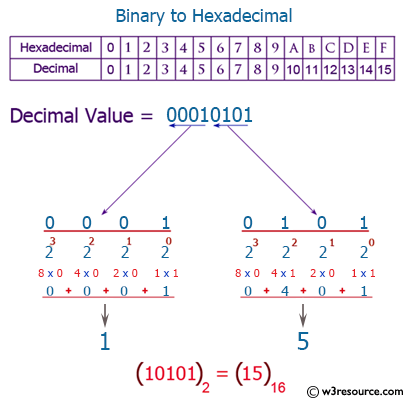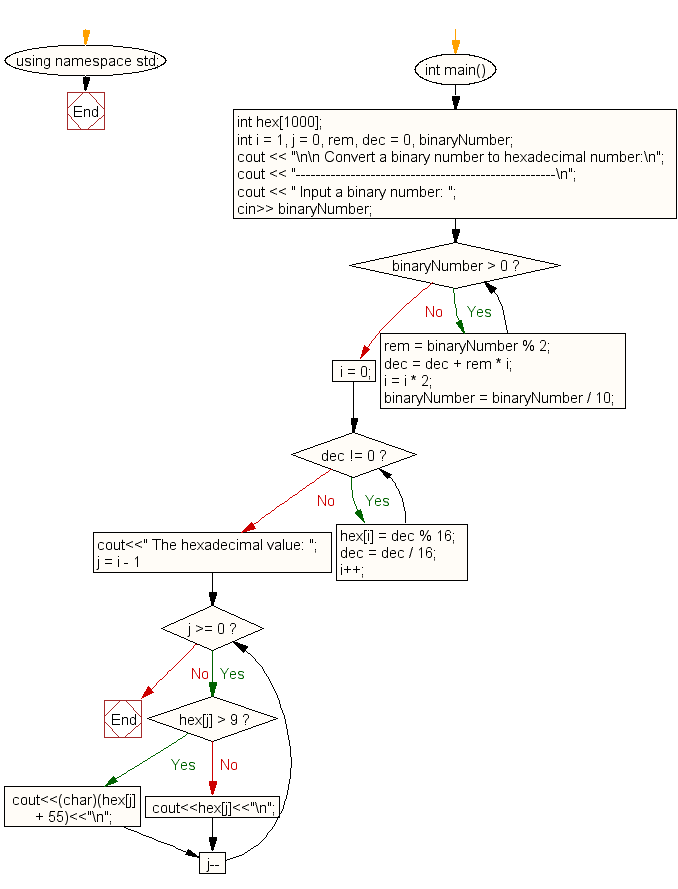﻿ C++ Exercises: Convert a binary number to hexadecimal number - w3resource

# C++ Exercises: Convert a binary number to hexadecimal number

## C++ For Loop: Exercise-74 with Solution

Write a program in C++ to convert a binary number to hexadecimal number.

Visual Presentation:Sample Solution:-

C++ Code :

``````#include <iostream> // Include input/output stream library
#include <math.h>   // Include math library for mathematical functions
using namespace std; // Use the standard namespace

int main() // Main function where the execution of the program starts
{
int hex; // Declare an array to store hexadecimal digits
int i = 1, j = 0, rem, dec = 0, binaryNumber; // Declare variables for iteration, remainders, decimal and binary numbers

cout << "\n\n Convert a binary number to hexadecimal number:\n"; // Display message prompting for binary to hexadecimal conversion
cout << "----------------------------------------------------\n"; // Display separator line
cout << " Input a binary number: "; // Prompt user to input a binary number
cin >> binaryNumber; // Read the binary number input by the user

while (binaryNumber > 0) // Loop to convert binary to decimal
{
rem = binaryNumber % 2; // Calculate the remainder by performing modulo 2 operation
dec = dec + rem * i; // Calculate the decimal number by adding the remainder multiplied by a factor 'i'
i = i * 2; // Multiply the factor 'i' by 2 for the next iteration
binaryNumber = binaryNumber / 10; // Update the binary number by removing its last digit (rightmost digit)
}

i = 0; // Reset the iteration variable to zero
while (dec != 0) // Loop to convert decimal to hexadecimal
{
hex[i] = dec % 16; // Calculate the remainder after dividing by 16
dec = dec / 16; // Update the decimal number by dividing by 16
i++; // Increment the index for the hexadecimal array
}

cout << " The hexadecimal value: "; // Display the resulting hexadecimal value
for (j = i - 1; j >= 0; j--) // Loop to display the hexadecimal digits
{
if (hex[j] > 9) // If the value is greater than 9, print corresponding character (A-F)
{
cout << (char)(hex[j] + 55) << "\n"; // Convert and display hexadecimal values to characters (A-F)
}
else // If the value is less than or equal to 9, print the value
{
cout << hex[j] << "\n"; // Display hexadecimal values as numbers
}
}
}
``````

Sample Output:

``` Convert a binary number to hexadecimal number:
----------------------------------------------------
Input a binary number: 1011
```

Flowchart:C++ Code Editor: